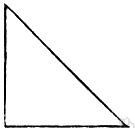# right-angled triangle

Also found in: Thesaurus, Medical, Financial, Encyclopedia, Wikipedia.
Related to right-angled triangle: hypotenuse

## right-angled triangle

n
(Mathematics) a triangle one angle of which is a right angle. US and Canadian name: right triangle
Collins English Dictionary – Complete and Unabridged, 12th Edition 2014 © HarperCollins Publishers 1991, 1994, 1998, 2000, 2003, 2006, 2007, 2009, 2011, 2014
ThesaurusAntonymsRelated WordsSynonymsLegend:
 Noun 1right-angled triangle - a triangle with one right angle  right triangletriangle, trigon, trilateral - a three-sided polygonhypotenuse - the side of a right triangle opposite the right angle
Based on WordNet 3.0, Farlex clipart collection. © 2003-2012 Princeton University, Farlex Inc.
References in periodicals archive ?
What is the longest side called on a right-angled triangle? 6.
WHO most famously gave his name to the theorem which determines the length of the side opposite the right angle in a right-angled triangle? WHAT may be civil, limited or total?
Extending two lines from extreme points A and B to meet any arbitrary point C in the circle always gives a right-angled triangle (Figure 3b).
What name is given to the side of a right-angled triangle opposite to the angle of 90 degrees?
The L-shaped slit S1 consists of two rectangular slits with lengths of [d.sub.1] and [d.sub.2] and is formed at the opposite side of the first right-angled triangle at a distance of [x.sub.1] from the left edge of the Spidron fractal slot.
Two of Euclid's theorems - 'of a leg' and 'of an altitude', which deal with the right-angled triangle, are given in  as predecessors of the Pythagoras' theorem in the following wording.
An isosceles right-angled triangle metallic patch with the two equal sides of [M.sub.x] = [M.sub.y] = 16 mm is printed on the upper side of the substrate.
In geometry, how many right angles does a right-angled triangle have?
Repeated applications of Pythagoras' Rule, relating the lengths of the sides of a right-angled triangle, can be used to find the relative distances between the centre and the vertices, and also the relative distances between vertices.
Al-Ward, born in Idleb province in 1971, said that the discovered laws produced a relation which specifies the connection between the sharp angles and sides in the right-angled triangle.
Then draw one large right-angled triangle and one smaller right-angled triangle.

Site: Follow: Share:
Open / Close